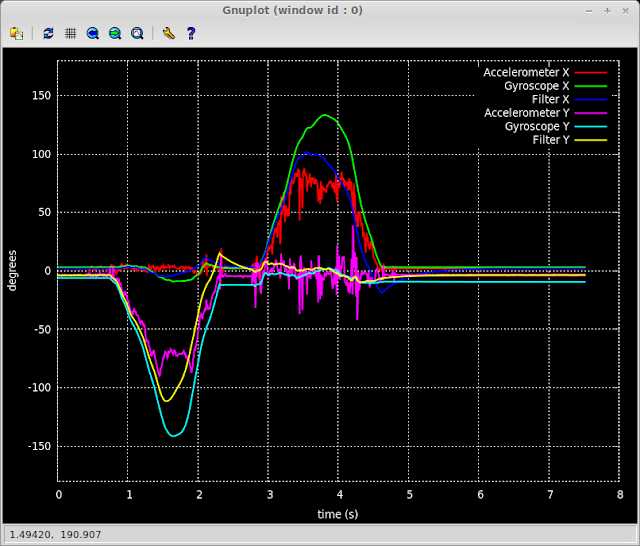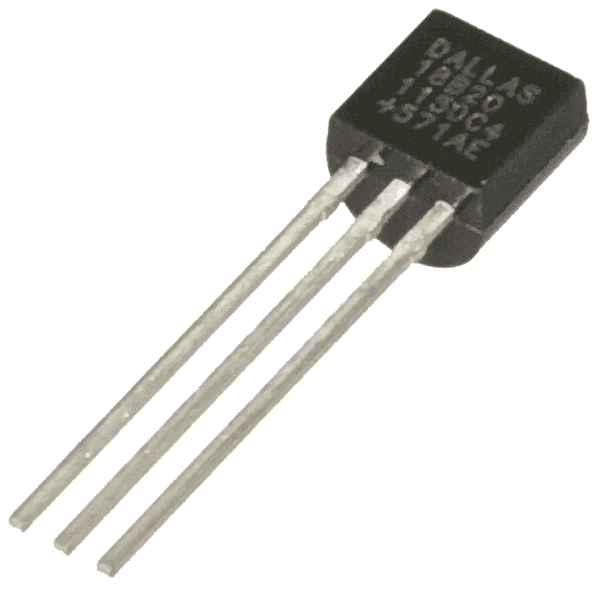## Saturday, 16 November 2013

### Using a complementary filter to combine Accelerometer and Gyroscopic data

This post shows how to combine data from the accelerometer and gyroscope using a complementary filter to produce a better readings from the MPU-6050.Complementary filter
The image above shows data for a negative rotation around the Y axis followed by a positive rotation around the X axis.  It includes the base accelerometer, gyroscope and the filtered data.  Lets look at things in a bit more detail.

The following graph show a simple rotation in X of roughly 90-100 degrees (I didn't measure it accurately).  The red line shows the accelerometer data and as we can see from the spikes it's a noisy data set.  The green line show the rotation angle calculated from summing the individual angles read from the gyroscope.  While this data is less noisy it is prone to drift over time, the gyroscope doesn't return back to zero when not moving.

The blue line shows the complementary filter at work.  It combines the two data sets by merging fast rotations from the gyroscope with the slower trends from the accelerometer and we get the best of both worlds. For a full explanation of the theory behind this type of filter I recommend reading this excellent paper The Balance Filter by Shane Colton.  If you just want some simple code then read on.

The interesting parts are lines 76 to 91.
``````#!/usr/bin/python

import smbus
import math
import time

# Power management registers
power_mgmt_1 = 0x6b
power_mgmt_2 = 0x6c

gyro_scale = 131.0
accel_scale = 16384.0

gyro_scaled_x = twos_compliment((raw_gyro_data << 8) + raw_gyro_data) / gyro_scale
gyro_scaled_y = twos_compliment((raw_gyro_data << 8) + raw_gyro_data) / gyro_scale
gyro_scaled_z = twos_compliment((raw_gyro_data << 8) + raw_gyro_data) / gyro_scale

accel_scaled_x = twos_compliment((raw_accel_data << 8) + raw_accel_data) / accel_scale
accel_scaled_y = twos_compliment((raw_accel_data << 8) + raw_accel_data) / accel_scale
accel_scaled_z = twos_compliment((raw_accel_data << 8) + raw_accel_data) / accel_scale

return (gyro_scaled_x, gyro_scaled_y, gyro_scaled_z, accel_scaled_x, accel_scaled_y, accel_scaled_z)

def twos_compliment(val):
if (val >= 0x8000):
return -((65535 - val) + 1)
else:
return val

def dist(a, b):
return math.sqrt((a * a) + (b * b))

def get_y_rotation(x,y,z):

def get_x_rotation(x,y,z):

bus = smbus.SMBus(0)  # or bus = smbus.SMBus(1) for Revision 2 boards

# Now wake the 6050 up as it starts in sleep mode

now = time.time()

K = 0.98
K1 = 1 - K

time_diff = 0.01

(gyro_scaled_x, gyro_scaled_y, gyro_scaled_z, accel_scaled_x, accel_scaled_y, accel_scaled_z) = read_all()

last_x = get_x_rotation(accel_scaled_x, accel_scaled_y, accel_scaled_z)
last_y = get_y_rotation(accel_scaled_x, accel_scaled_y, accel_scaled_z)

gyro_offset_x = gyro_scaled_x
gyro_offset_y = gyro_scaled_y

gyro_total_x = (last_x) - gyro_offset_x
gyro_total_y = (last_y) - gyro_offset_y

print "{0:.4f} {1:.2f} {2:.2f} {3:.2f} {4:.2f} {5:.2f} {6:.2f}".format( time.time() - now, (last_x), gyro_total_x, (last_x), (last_y), gyro_total_y, (last_y))

for i in range(0, int(3.0 / time_diff)):
time.sleep(time_diff - 0.005)

(gyro_scaled_x, gyro_scaled_y, gyro_scaled_z, accel_scaled_x, accel_scaled_y, accel_scaled_z) = read_all()

gyro_scaled_x -= gyro_offset_x
gyro_scaled_y -= gyro_offset_y

gyro_x_delta = (gyro_scaled_x * time_diff)
gyro_y_delta = (gyro_scaled_y * time_diff)

gyro_total_x += gyro_x_delta
gyro_total_y += gyro_y_delta

rotation_x = get_x_rotation(accel_scaled_x, accel_scaled_y, accel_scaled_z)
rotation_y = get_y_rotation(accel_scaled_x, accel_scaled_y, accel_scaled_z)

last_x = K * (last_x + gyro_x_delta) + (K1 * rotation_x)
last_y = K * (last_y + gyro_y_delta) + (K1 * rotation_y)

print "{0:.4f} {1:.2f} {2:.2f} {3:.2f} {4:.2f} {5:.2f} {6:.2f}".format( time.time() - now, (rotation_x), (gyro_total_x), (last_x), (rotation_y), (gyro_total_y), (last_y))
``````
First we read all the scaled data from the device.
``````    (gyro_scaled_x, gyro_scaled_y, gyro_scaled_z, accel_scaled_x, accel_scaled_y, accel_scaled_z) = read_all()
``````
Adjust the gyroscope data by the offset.
``````    gyro_scaled_x -= gyro_offset_x
gyro_scaled_y -= gyro_offset_y
``````
The offset is the value of the gyroscope reading when it's not moving and is taken from the very first reading. This is fine for simple testing but ideally a true offset value should be determined by calibrating the sensor.
Now calculate the gyroscope delta, this is how much the sensor has rotated since the last sample was taken and then add it to a running total
``````    gyro_x_delta = (gyro_scaled_x * time_diff)
gyro_y_delta = (gyro_scaled_y * time_diff)

gyro_total_x += gyro_x_delta
gyro_total_y += gyro_y_delta
``````
This gives us a rotation angle just from reading from the gyroscope (the green line in the graph above)
Next read the rotation values from the accelerometer just like we did in the previous post
``````    rotation_x = get_x_rotation(accel_scaled_x, accel_scaled_y, accel_scaled_z)
rotation_y = get_y_rotation(accel_scaled_x, accel_scaled_y, accel_scaled_z)
``````
Now the complementary filter is used to combine the data.
``````    last_x = K * (last_x + gyro_x_delta) + (K1 * rotation_x)
last_y = K * (last_y + gyro_y_delta) + (K1 * rotation_y)
``````
We take the previous readings (last_x, last_y) and add in the gyroscope data then scale this by K, then add in the accelerometer data scaled by K1 and this value is our new angle. The coefficients K and K1 should add up to 1, in this case they are 0.98 and 0.02 respectively. You can change the values of K and K1 to suit your application as described in the previously linked article. The time intervals for the loop needs to be reasonably accurate for this to work well and the sample rate should be 100Hz or higher.

If you run the code and direct the output to a file
```    sudo ./filter-test.py > plot.dat
```
you can then generate gnuplot diagrams similar to those above, save the following to a file gnuplot-command.plg
```    set terminal wxt persist size 800,600 background '#000000' # enhanced font 'Consolas,10'

set style line 99 linecolor rgb "#ffffff" linetype 0 linewidth 2
set key top right textcolor linestyle 99
set grid linestyle 99
set border linestyle 99

set xlabel "time (s)" textcolor linestyle 99
set ylabel "degrees" textcolor linestyle 99

set yrange [-180:180]

plot filename using 1:2 title "Accelerometer X" with line linewidth 2 , \
filename using 1:3 title "Gyroscope X" with line linewidth 2 , \
filename using 1:4 title "Filter X" with line linewidth 2

plot filename using 1:5 title "Accelerometer Y" with line linewidth 2 , \
filename using 1:6 title "Gyroscope Y" with line linewidth 2 , \
filename using 1:7 title "Filter Y" with line linewidth 2
```
then to generate a graph
```    gnuplot -e "filename='plot.dat'" gnuplot-command.plg
```

In the next post I show how to hook up a HMC5883L Compass module and incorporate it into the code so we can get a true bearing.  Well that is when I get a new one as I seem to have fried mine.